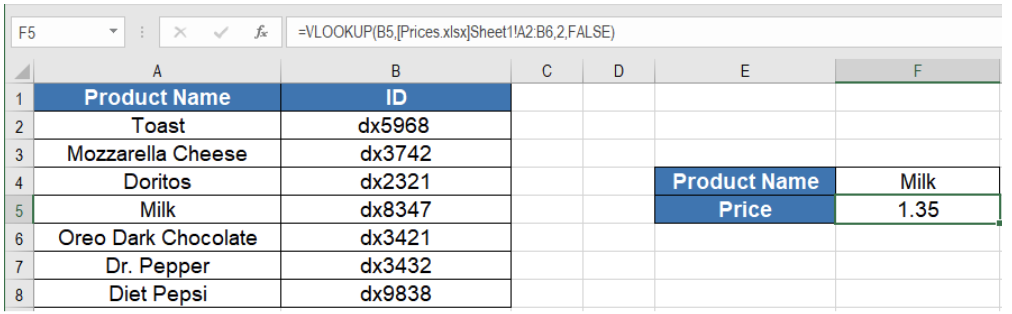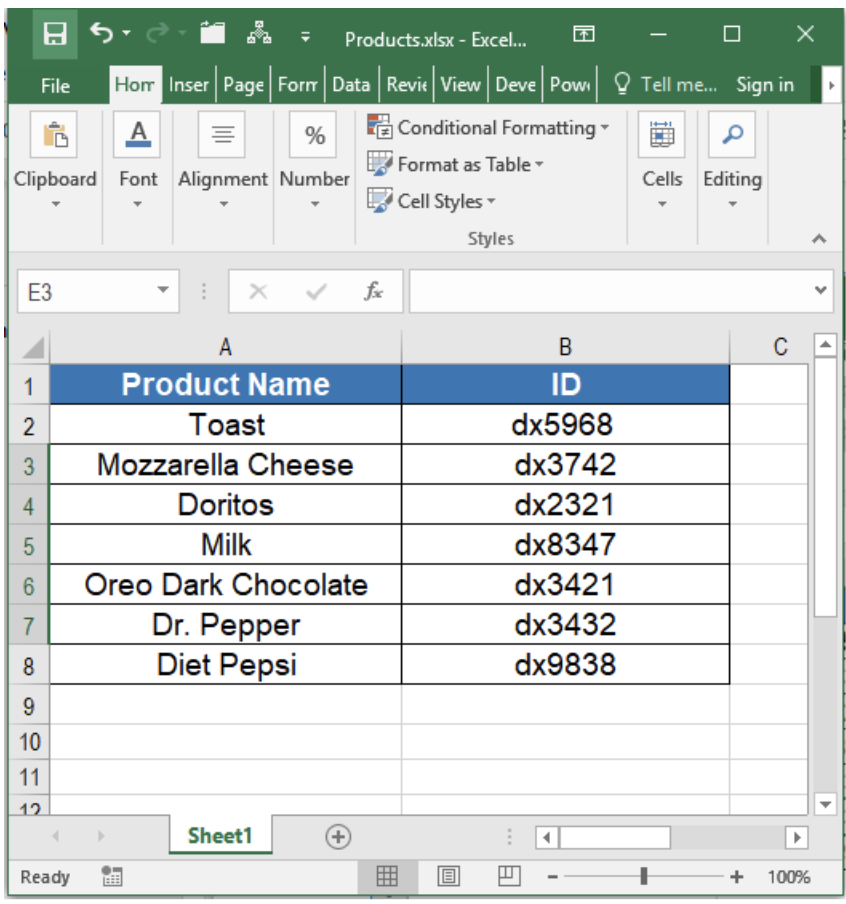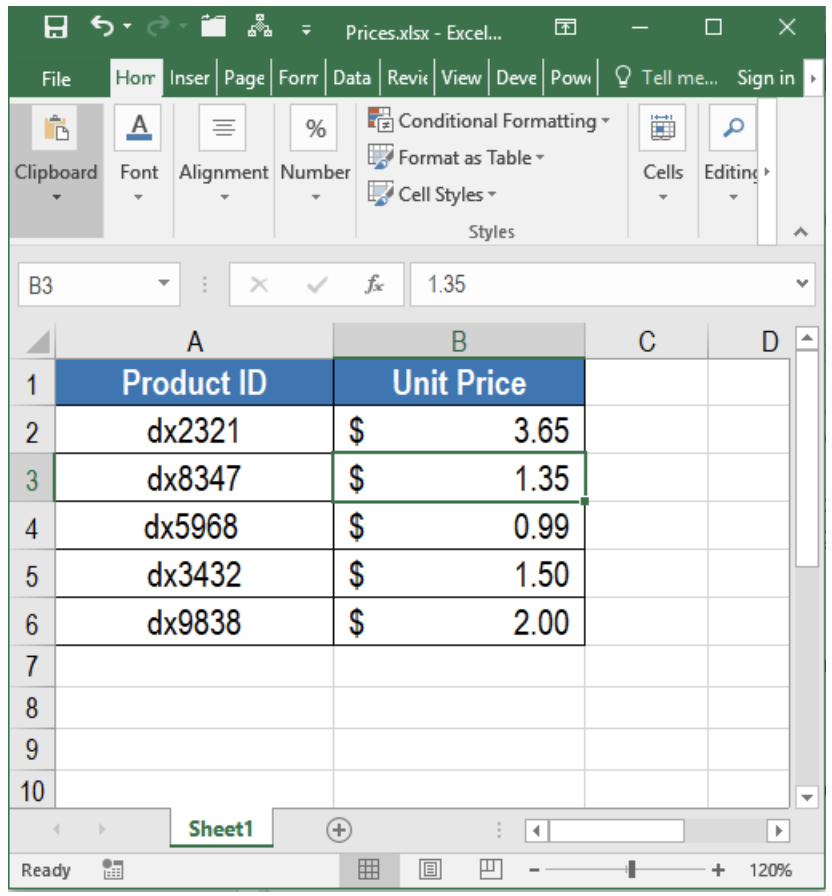Get instant live expert help with Excel or Google Sheets“My Excelchat expert helped me in less than 20 minutes, saving me what would have been 5 hours of work!”

#### Post your problem and you’ll get Expert help in seconds.

Your message must be at least 40 characters
Our professional Expert are available now. Your privacy is guaranteed.

# How to use VLOOKUP with Multiple Workbooks

VLOOKUP is one of the primary function used for lookup and reference in Excel. One of the many limitations of VLOOKUP is its inability to work with multiple workbooks. This tutorial will walk through how to VLOOKUP with multiple workbooks.Figure 1. Example of How to Use VLOOKUP with Multiple Workbooks

## The Generic Formula to use VLOOKUP across Multiple Workbooks

`=VLOOKUP(lookup_value ,[workbook]sheet!range,col_num,match_type)`

## Setting up Data

The following example consists of a product sales data set. It has two different workbooks to store the product information. The first workbook Products.xlsx has the product name and Ids.Figure 2. Product Information Workbook for VLOOKUP with Multiple Workbooks

The second workbook Prices.xlsx has the prices for the corresponding IDs. We can find the price for Milk in the first workbook using VLOOKUP with a reference to the second workbook.Figure 3. Product Price Workbook for VLOOKUP with Multiple Workbooks

Let us look at an example on how to find the sales of milk using VLOOKUP across these workbooks. To do that we need to:

• Go to cell F5.
• Assign the formula =VLOOKUP(B5,[Prices.xlsx]Sheet1!A2:B6,2,FALSE).
• Press Enter to apply it to F5.

This will return the sales for milk in F5 using the two workbooks. The VLOOKUP formula helps to look up for the ID for milk in the prices workbook.

VLOOKUP is the most helpful function for lookup operations. VLOOKUP fails to perform lookup across multiple workbooks in its general format. But we learned in this tutorial how to change it to use VLOOKUP with multiple workbooks.

## Instant Connection to an Expert through our Excelchat Service

Most of the time, the problem you will need to solve will be more complex than a simple application of a formula or function. If you want to save hours of research and frustration, try our live Excelchat service! Our Excel Experts are available 24/7 to answer any Excel question you may have. We guarantee a connection within 30 seconds and a customized solution within 20 minutes.

### Did this post not answer your question? Get a solution from connecting with the expert.Another blog reader asked this question today on Excelchat:
Solution examplesI have a pivot table in which per order (on the rows) stands how much products they ordered per size (on the columns). I want to determine which combinations of quantities of sizes people order. And I want to count these combinations.
Solved by E. W. in 60 minsI have 500 numbers in column A with aproximatly 15digits. In column B I have 5 numbers with 6 digits Can a find a formula wich can transfer all numbers from A column wich start with some 6 digitsa from B column
Solved by V. L. in 32 minsI have a sheet with 3 columns. First column is a code for records in column B (has 563 records). What I need is a formula to show me if what is in column C (has 4400 records) is in column B and if it is to take the code for that record. Example: Column A(code corresponding to column B): 12, 14, 15, 19 Column B(names): asd, adf, ade, aqw Column C(names): akd, adf, ade, anb, wgs I need something that would say, if record in column C (for example adf) is matching record in column B (adf) return the code from column A (the code corresponding to that record in column B),if not return blank.
Solved by C. J. in 8 minsIf the data in A matches the data in C, then I need the data in E to be placed in B
Solved by X. W. in 40 minsI have a workbook with 2 sheets. First sheet has column A which is a device name, then columns B - infinity that have numeric "tags" for that device. Each device can have one or 20 "tags", tags are all numerical. Worksheet 2 has column A which is numeric value and B which is a human readable value of what the tag is. Question is, how do I replace all the tag numbers in worksheet 1 with the actual human readable values is sheet 2?
Solved by T. H. in 16 mins## Subscribe to Excelchat.coAnother blog reader asked this question today on Excelchat: